Function Repository Resource:

# FrobeniusDSolveFormula

Compute the formula for the Frobenius series solution to an ODE

Contributed by: Rauan Kaldybayev
 ResourceFunction["FrobeniusDSolveFormula"][eqn,u,x,x0] outputs a formula to be fed into the resource function FrobeniusDSolve. ResourceFunction["FrobeniusDSolveFormula"][eqn,u,x,x0,Association] outputs the formula for the Frobenius series solution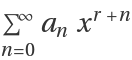of a differential equation for the function u, with independent variable x.

## Details and Options

The output is a formula describing a Frobenius series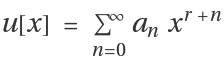. It is a list of the form {elem1,elem2,}, where the elemi gives information about the ith solution.
Solving ODEs using Frobenius's method is a tedious monotonous work, yet it is very simple - a perfect process to automate. ResourceFunction["FrobeniusDSolveFormula"] can be used to compute a formula for the Frobenius series solution to an ODE. Computing a Frobenius series solution by hand can lead to potential insights or be helpful in conceptually understanding the behavior of the solutions.
Another application for ResourceFunction["FrobeniusDSolveFormula"] is to assist the resource function FrobeniusDSolve. When solving an ODE, FrobeniusDSolve first utilizes ResourceFunction["FrobeniusDSolveFormula"] to compute a formula for the solution. The formula is then used to calculate the approximate solutions. When a single ODE is to be solved many times with different parameters, computation time can be significantly reduced by computing the formula separately, using ResourceFunction["FrobeniusDSolveFormula"]. (see Applications)
The function can be used to solve linear homogeneous ODEs with polynomial coefficients.
Any linear ODE with polynomial coefficients can be written in the form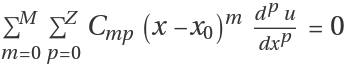for some (any) x0. Frobenius's method assumes solution of the form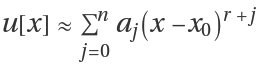; substituting this into the equation yields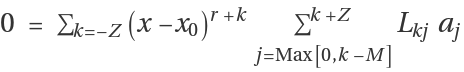, where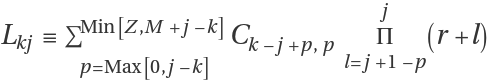. To satisfy the equation, the coefficients before all powers of x-x0 must be zero for all k. From here, we can determine the possible values of the constant r for which a00, and then for each r, compute the coefficients. Read more at the related Wolfram Community post.
The options for ResourceFunction["FrobeniusDSolveFormula"] are:
 "ComputeError" False whether to compute the error of the approximate solution

## Examples

### Basic Examples (2)

Obtain a formula for the Frobenius solution for the ODE 2x2y''+7x(x+1)y'-3y=0 near the singular point x=0:

 In:=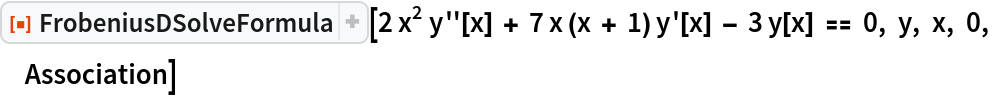Out=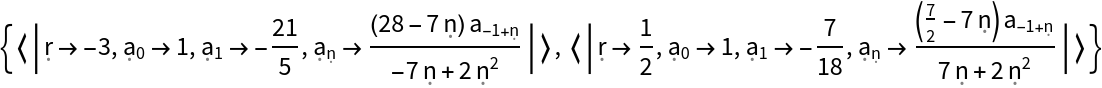The formula describes a Frobenius series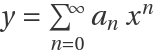. Display the same formula in a form compatible with the resource function FrobeniusDSolve (see Properties and Relations):

 In:=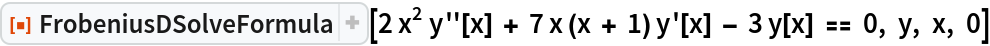Out=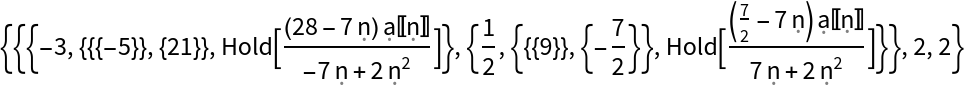### Scope (3)

The function can operate with complex numbers:

 In:=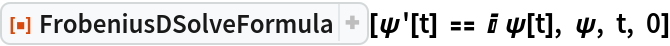Out=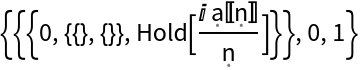The inputs of the function can be symbolics:

 In:=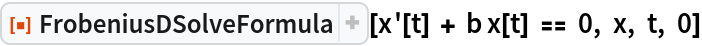Out=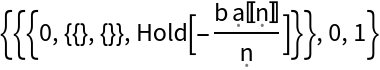FrobeniusDSolveFormula can be used to solve ODEs of any order. Here is the formula for the Frobenius series solution to (x-1)4f''''[x]=x(2+x)f[x]+x2(x-1)2f''[x]-19(x-1)3f'''[x] near the singular point x=1:

 In:=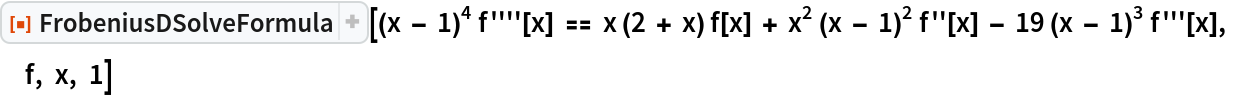Out=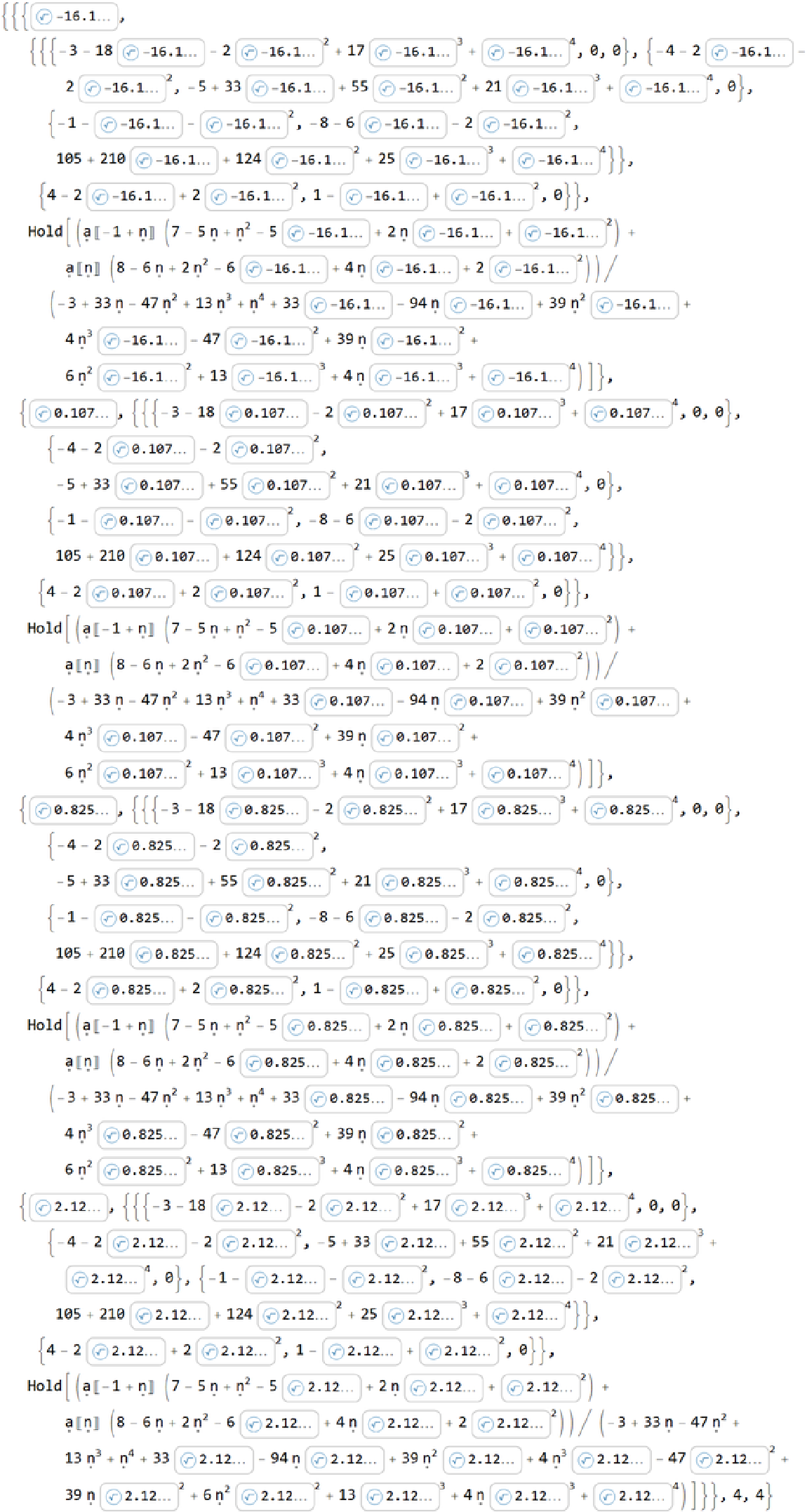### Options (1)

If the option "ComputeError" is set to True, FrobeniusDSolveFormula will not only output a formula for the Frobenius series solution but also the formula for the error of this solution:

 In:=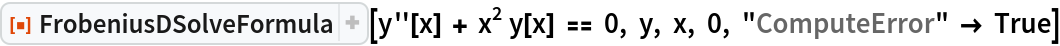Out=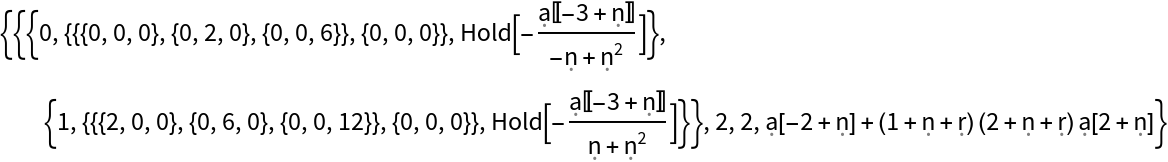The formula doesn't make much sense to a human and instead is meant to be fed into the resource function FrobeniusDSolve, which will in turn compute an approximate solution of the form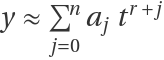and the error of this solution.

### Applications (2)

FrobeniusDSolve is a related resource function that computes approximate Frobenius and power series solutions to ODEs. The solutions it outputs are of the form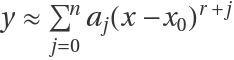for some parameters x0, j. The following is an example of how FrobeniusDSolve can be used to obtain approximate solutions to the ODE x2y''-2xy'+(1+x)y=0 with x0=0 and n=4:

 In:=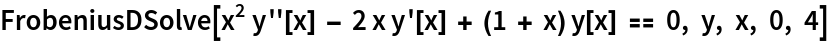Out=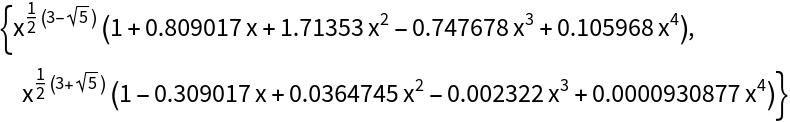When solving an ODE, FrobeniusDSolve first utilizes FrobeniusDSolveFormula to compute a formula for the solution. The formula is then used to calculate the approximate solutions. When a single ODE is to be solved many times with different parameters, computation time can be significantly reduced by computing the formula separately, using FrobeniusDSolveFormula. This way, FrobeniusDSolve doesn't have to compute the formula every time, and it only has to do the numerical part of the computation. As can be seen from the following example, the decrease in computation time is dramatic:

 In:=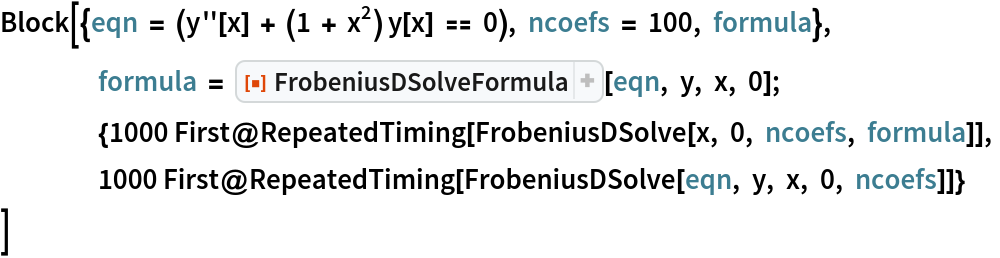Out=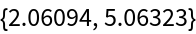### Properties and Relations (2)

Formulas given by FrobeniusDSolveFormula can be fed into the resource function FrobeniusDSolve to compute approximate Frobenius series solutions. This can help significantly improve efficiency when a single ODE is to be solved repeatedly (see Applications). Obtain a formula for the Frobenius solution for the ODE 2x2y''+7x(x+1)y'-3y=0 near the singular point x=0, and output it in a form compatible with FrobeniusDSolve:

 In:=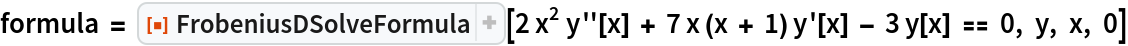Out=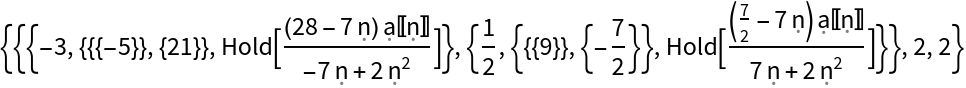Use the formula to compute two linearly independent approximate solutions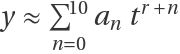:

 In:=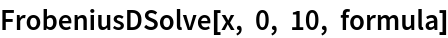Out=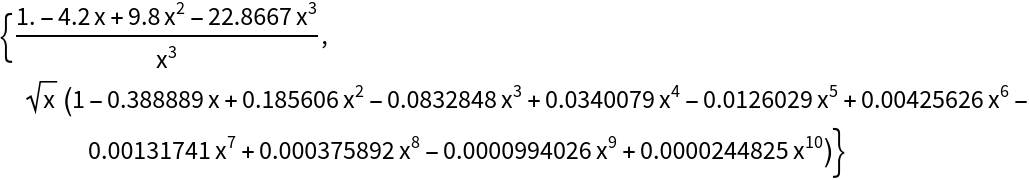In:=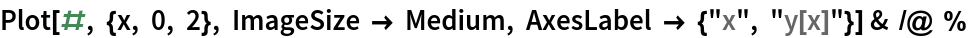Out=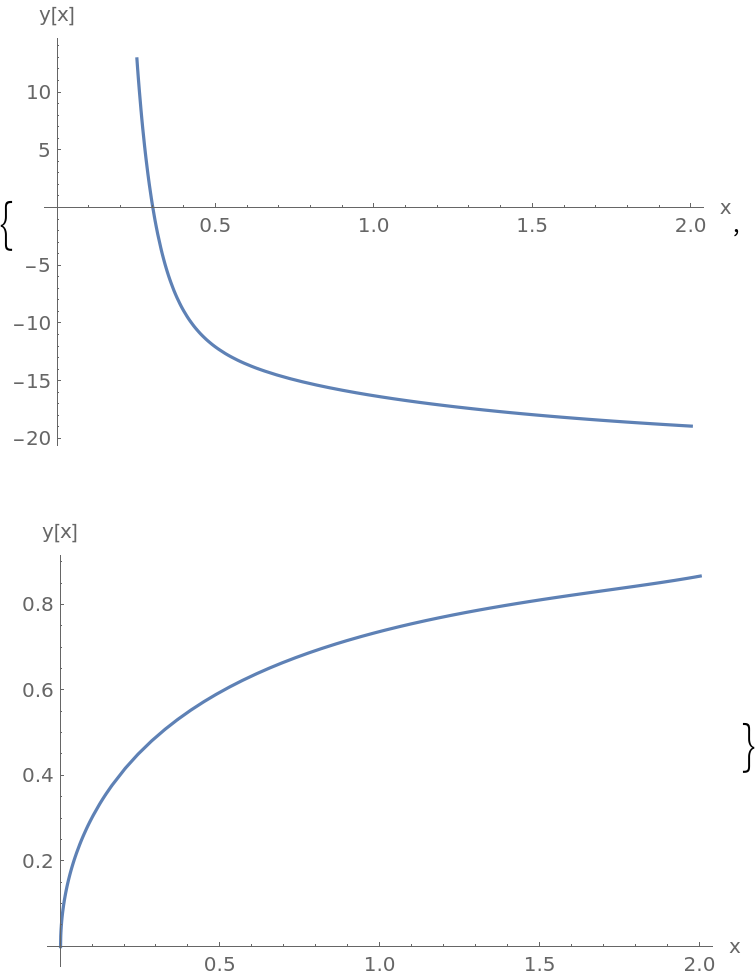### Possible Issues (2)

The ODE must be linear and homogeneous, and its coefficients must be polynomial. Otherwise, FrobeniusDSolveFormula returns \$Failed:

 In:=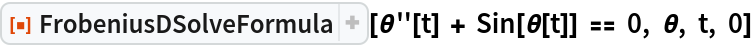Out=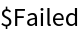In:=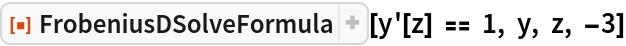Out=In:=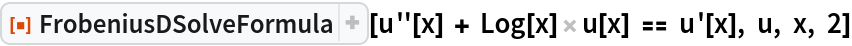Out=If the ODE isn't liner but can be made so by dividing by a non-zero factor, the function still doesn't accept it:

 In:=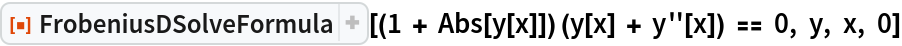Out=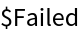## Publisher

Wolfram Summer Camp

## Version History

• 1.0.0 – 13 April 2021